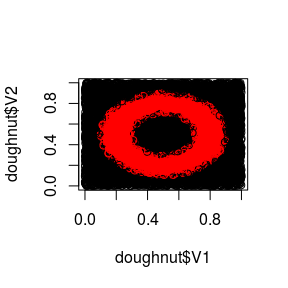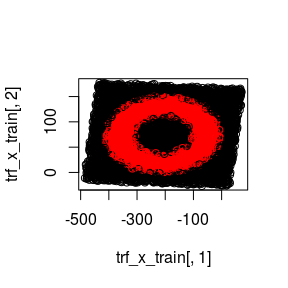# nntrf package

#### 2021-02-26

library(dplyr)
#>
#> Attaching package: 'dplyr'
#> The following objects are masked from 'package:stats':
#>
#>     filter, lag
#> The following objects are masked from 'package:base':
#>
#>     intersect, setdiff, setequal, union
library(tidyr)
library(ggplot2)
library(ggridges)
#>
#> Attaching package: 'ggridges'
#> The following object is masked from 'package:ggplot2':
#>
#>     scale_discrete_manual
library(nntrf)

# Usage

nntrf stands for Neural Net Transformation. The aim of this package is to use the hidden layer weights of a neural network (NN) as a transformation of the dataset, that can be used by other machine learning methods.

Mathematically, a standard NN with one hidden layer is $$\hat{y} = S(S(x*W_1)*W_2)$$, where $$x$$ is one instance ($$x = (1, x_1, x_2, x_n)$$) and $$W_1$$ and $$W_2$$ are the weights of the hidden and output layer (including the biases), respectively ($$*$$ is the matrix product and $$S()$$ is the sigmoid function). The aim of nntrf is to train a NN with some training data $$(X,Y)$$ and then use $$W_1$$ to transform datasets via $$X' = S(X*W_1)$$. Obviously, the same transformation can be applied to test datasets. This transformation is supervised, because a NN was trained to approximate this problem, as opposed to unsupervised transformations like PCA.

In order to show how this can be used, the doughnut dataset will be used. It is a two-class problem: black and red.

data("doughnut")
plot(doughnut$V1, doughnut$V2, col=doughnut$V3)The doughnut dataset has been altered by adding 8 random features (uniform noise between 0 and 1) and performing a random rotation on the resulting dataset. The result is the doughnutRandRotated dataset with 10 features. head(doughnutRandRotated,5) #> V1 V2 V3 V4 V5 V6 #> 89670 0.6021107 0.7512063 0.6413899 0.5535337 0.5390535 0.5525395 #> 26551 0.7731719 0.5842861 0.5691011 0.3904659 0.7253717 0.6154807 #> 37212 0.4344544 0.4649177 0.4486301 0.5768168 0.6470240 0.3402623 #> 57284 0.6106970 0.3638407 0.3321711 0.7234145 0.5072863 0.2877618 #> 90818 0.5984079 0.5049844 0.6747645 0.5625673 0.5348219 0.7918175 #> V7 V8 V9 V10 V11 #> 89670 0.5227248 0.4539889 0.5757919 0.8562990 FALSE #> 26551 0.6651487 0.4735743 0.4110602 0.3961613 TRUE #> 37212 0.5905573 0.3243177 0.4243501 0.5414516 TRUE #> 57284 0.4236246 0.2953086 0.5131089 0.4344240 FALSE #> 90818 0.5558537 0.6074310 0.6909910 0.8096290 FALSE The goal of nntrf here is to recover the original dataset. The process is similar to the nntrf::nntrf_doughnut() function, but it has been repeated in the following R code for illustration purposes. A NN with 4 hidden neurons and 100 iterations is used. knn (with 1 neighbor) will be used to assess the quality of the transformation. knn is a lazy machine learning method. It does not construct a model, but rather relies on the data to classify new instances. It is known that knn does not behave well when dimensionality is high, or when there are many irrelevant or redundant attributes. Therefore, it is a good choice to evaluate the quality of the features generated by nntrf. We can see that the success rate of knn improves after the nntrf transformation. Notice that for this problem the transformation $$X' = X*W_1$$ (use_sigmoid=FALSE) works better than $$X' = S(X*W_1)$$ (use_sigmoid=TRUE). data("doughnutRandRotated") rd <- doughnutRandRotated rd$V11 <- as.factor(rd$V11) n <- nrow(rd) set.seed(0) training_index <- sample(1:n, round(0.6*n)) train <- rd[training_index,] test <- rd[-training_index,] x_train <- train[,-ncol(train)] y_train <- train[,ncol(train)] x_test <- test[,-ncol(test)] y_test <- test[,ncol(test)] set.seed(0) outputs <- FNN::knn(x_train, x_test, factor(y_train)) success <- mean(outputs == y_test) cat(paste0("Success rate of KNN (K=1) with doughnutRandRotated ", success, "\n")) #> Success rate of KNN (K=1) with doughnutRandRotated 0.60275 set.seed(0) nnpo <- nntrf(formula=V11~., data=train, size=4, maxit=100, trace=FALSE) # With sigmoid trf_x_train <- nnpo$trf(x=x_train,use_sigmoid=TRUE)
trf_x_test <- nnpo$trf(x=x_test,use_sigmoid=TRUE) outputs <- FNN::knn(trf_x_train, trf_x_test, factor(y_train)) success <- mean(outputs == y_test) cat(paste0("Success rate of KNN (K=1) with doughnutRandRotated transformed by nntrf with Sigmoid ", success, "\n")) #> Success rate of KNN (K=1) with doughnutRandRotated transformed by nntrf with Sigmoid 0.76775 # With no sigmoid trf_x_train <- nnpo$trf(x=x_train,use_sigmoid=FALSE)
trf_x_test <- nnpo$trf(x=x_test,use_sigmoid=FALSE) outputs <- FNN::knn(trf_x_train, trf_x_test, factor(y_train)) success <- mean(outputs == y_test) cat(paste0("Success rate of KNN (K=1) with doughnutRandRotated transformed by nntrf with no sigmoid ", success, "\n")) #> Success rate of KNN (K=1) with doughnutRandRotated transformed by nntrf with no sigmoid 0.964 Interestingly, attributes 1 and 2 of the transformed dataset have recovered the doughnut to some extent. plot(trf_x_train[,1], trf_x_train[,2], col=y_train)In some cases, NN training may get stuck in local minima. Parameter repetitions (with default = 1) allows to repeat the training process several times and keep the best NN in training. Next code shows an example with 5 repetitions, which in this case, improves slightly the previous results. set.seed(0) nnpo <- nntrf(repetitions=5, formula=V11~., data=train, size=4, maxit=100, trace=FALSE) trf_x_train <- nnpo$trf(x=x_train,use_sigmoid=FALSE)
trf_x_test <- nnpo$trf(x=x_test,use_sigmoid=FALSE) outputs <- FNN::knn(trf_x_train, trf_x_test, factor(y_train)) success <- mean(outputs == y_test) cat(paste0("Success rate of KNN (K=1) with doughnutRandRotated transformed by nntrf ", success, "\n")) #> Success rate of KNN (K=1) with doughnutRandRotated transformed by nntrf 0.9675 Important: The number of iterations and number of hidden neurons have been given some actual values as an example. But they are hyper-parameters that should be selected by means of hyper-parameter tuning. Packages such as MLR could help in this case. Next, nntrf is tried on iris, a 3-class classification problem. It can be seen that the 4-feature iris domain is transformed into a 2-feature domain, by means of nntrf, maintaining the success rate obtained with knn and the original dataset. rd <- iris n <- nrow(rd) set.seed(0) training_index <- sample(1:n, round(0.6*n)) train <- rd[training_index,] test <- rd[-training_index,] x_train <- as.matrix(train[,-ncol(train)]) y_train <- train[,ncol(train)] x_test <- as.matrix(test[,-ncol(test)]) y_test <- test[,ncol(test)] set.seed(0) outputs <- FNN::knn(x_train, x_test, train$Species)
success <- mean(outputs == test$Species) cat(paste0("Success rate of KNN (K=1) with iris ", success, "\n")) #> Success rate of KNN (K=1) with iris 0.966666666666667 set.seed(0) nnpo <- nntrf(formula = Species~., data=train, size=2, maxit=100, trace=FALSE) trf_x_train <- nnpo$trf(x=x_train,use_sigmoid=FALSE)
trf_x_test <- nnpo$trf(x=x_test,use_sigmoid=FALSE) outputs <- FNN::knn(trf_x_train, trf_x_test, train$Species)
success <- mean(outputs == test\$Species)
cat(paste0("Success rate of KNN (K=1) with iris transformed by nntrf ", success, "\n"))
#> Success rate of KNN (K=1) with iris transformed by nntrf 0.983333333333333